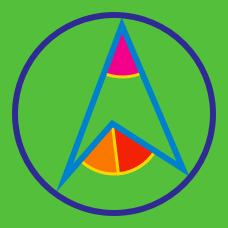Geometry

# Circle Properties: Level 3 Challenges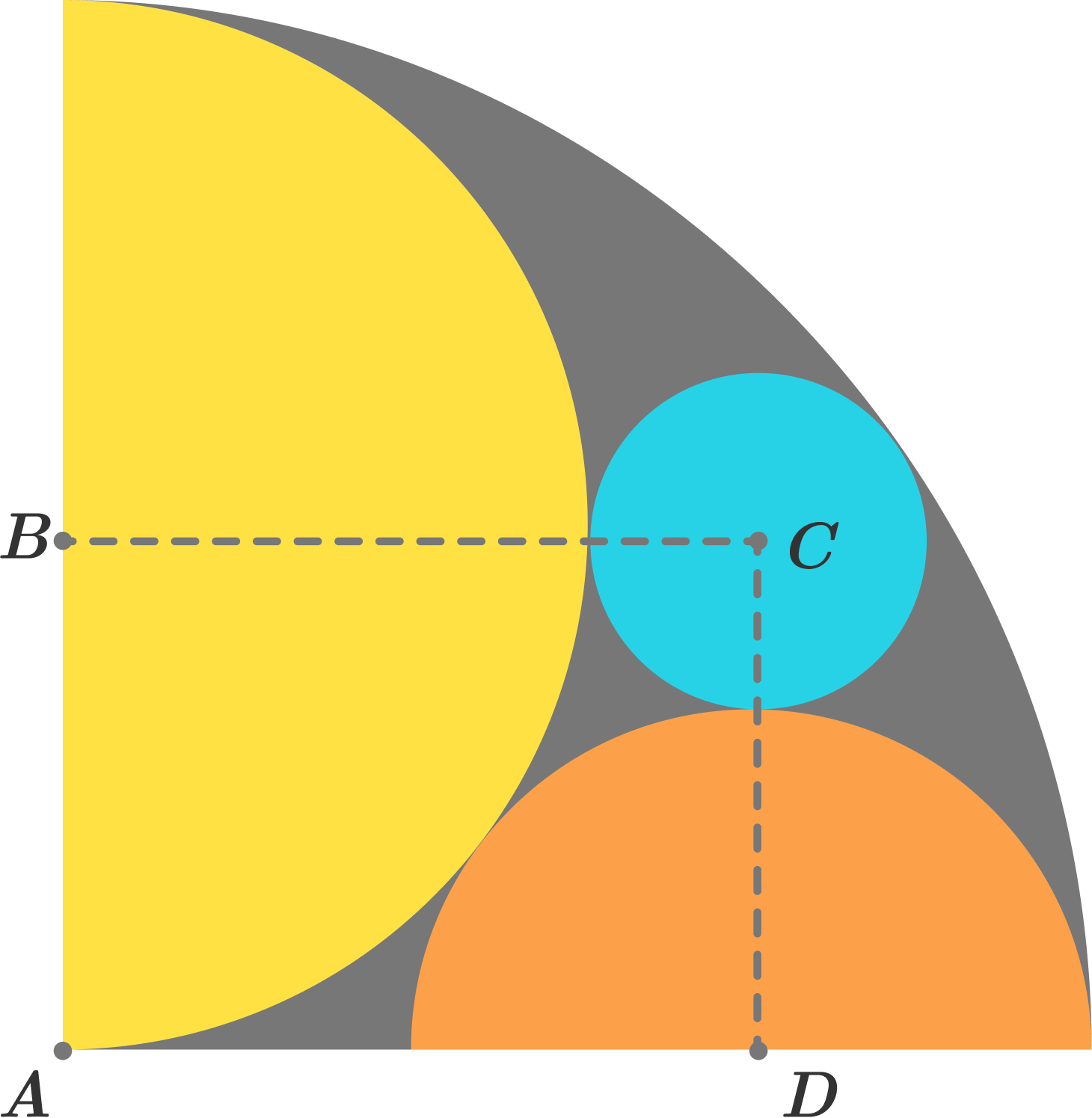A quarter-circle with radius $R$ is drawn. Inside it, two semicircles and a circle are drawn as shown in the above figure. If the area of the grey colored region is $\frac { \pi { R }^{ 2 } }{ X },$ find the value of $X.$$\Delta ABC$ is inscribed in a circle such that $864 \angle A = \angle B = \angle C$. If $B$ and $C$ are adjacent vertices of a regular $n$-gon inscribed in the circle, find $n$.

Details and Assumptions

• The diagram above is (extremely) not to scale.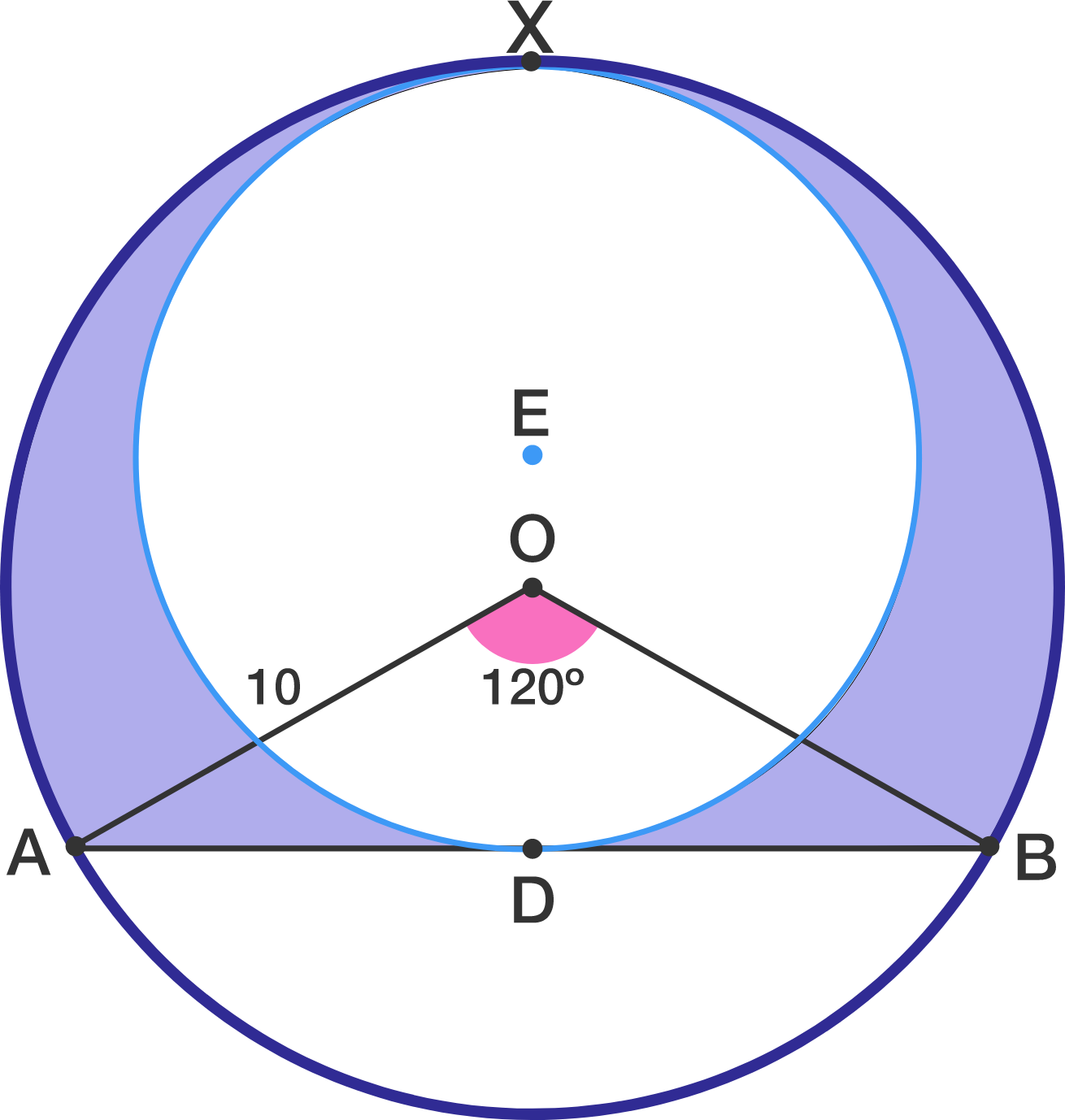In a circle with center $O$, a chord $AB$ is drawn such that $\angle AOB = 120^\circ$. Radius $AO=10$. A circle is drawn in the major arc such that it's radius is maximum, with center E, it touches the larger circle at point X as shown in figure.

The area of the shaded region can be expressed as $\dfrac{a\pi +b\sqrt{3}}{c}$ for integers $a,b,c$ with $\gcd(a,c)=1$ What is the value of $a+b+c$?

You are a farmer, with a round fenced field of radius $10$ meters. You tie your goat to the fence with a rope, and realize that the goat can eat exactly $\frac{1}{2}$ of the field. How long is the rope?

Note: You can use a computational solver for the last step of the problem.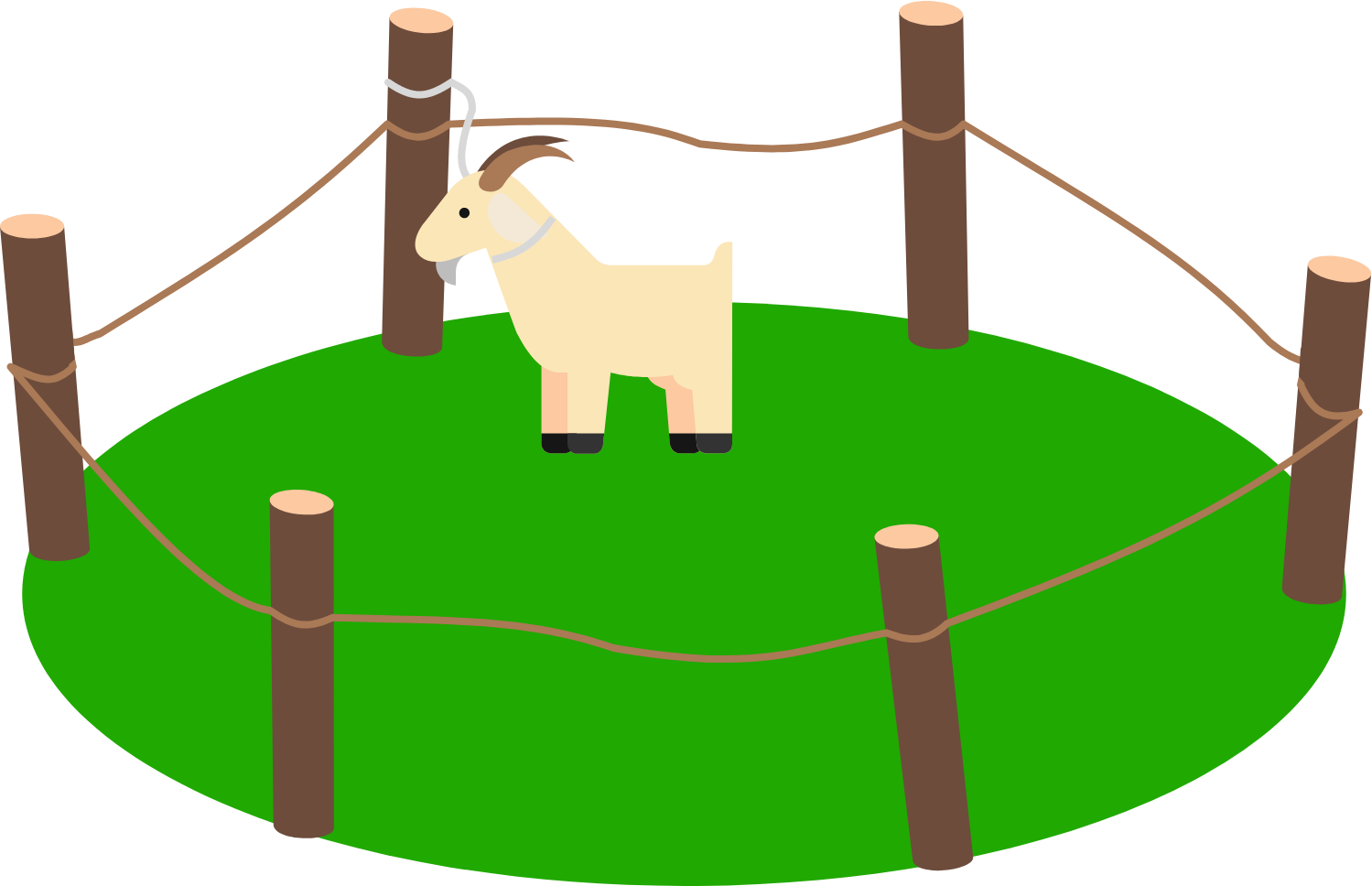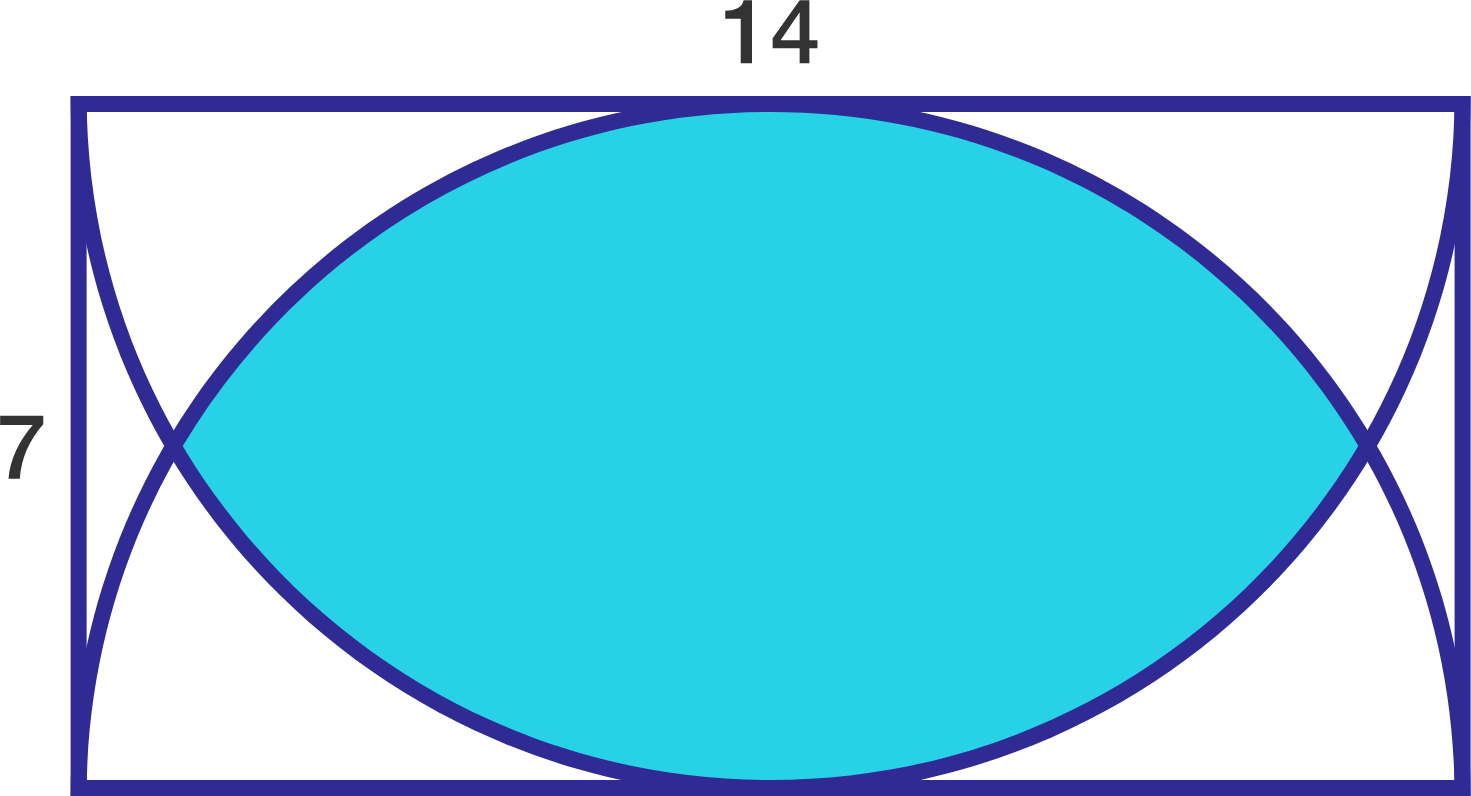Find the area of the blue shaded region in this $14\times 7$ rectangle, with two semicircles of radius 7 drawn.

×

Problem Loading...

Note Loading...

Set Loading...